# 升學解碼

## 留學美國「倒數」家長如何部署 第八課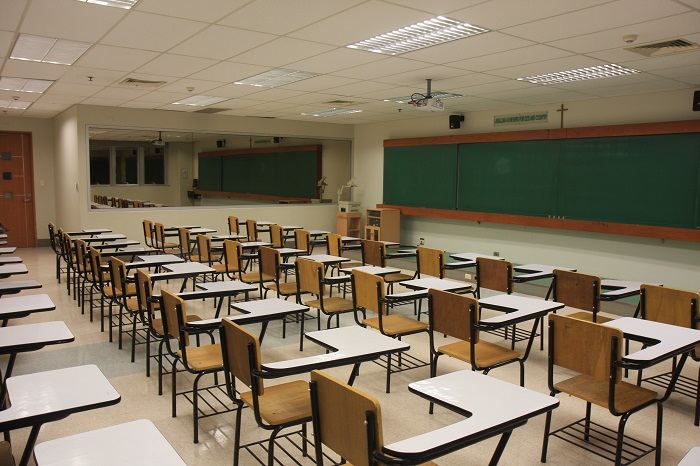## 美國學制高中GPA成績是怎麽計算的？

GPA 全稱 Grade Point Average，顧名思義，就是一個學生在某個階 段所選各門課程的成績平均數。對於將要申請大學的美國學制高中生來 說，GPA 就是其高中四年裏所有課程成績的平均數。

 A+ = 4.0 = 97-100 B+ = 3.3 = 87-89 C+ = 2.3 = 77-79 D+ = 1.3 = 67-69 A = 4.0 = 94-96 B = 3.0 = 84-86 C = 2.0 = 74-76 D- = 0.7 = 60-63 A- = 3.7 = 90-93 B- = 2.7 = 80-83 C- = 1.7 = 70-73 F = 0.0 = 0-59「讀寫障礙」少年到海外升學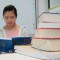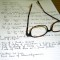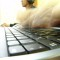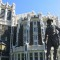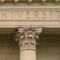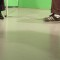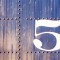《劃出生涯路》 生涯規劃 - 職業性向探索系列
STEM 創科教育之升學路
STEM 創科教育之生涯規劃

GCE A level 成績能否直接申請美國大學？
GCE A-Level 成績 可兌換在校學分